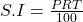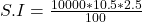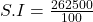## Sonia took a loan of $10 000 from ABC bank tob pay for a renovation at home. The bank offered her a period of 30 months at a rate of 10.5%. Question Sonia took a loan of$10 000 from ABC bank tob pay for a renovation at home. The bank offered her a period of 30 months at a rate of 10.5%. to repay the loan:

a) Calculate the Simple Interest she would pay in 30 months.

b) Calculate the Total amount Sonia would have to repay the bank.

in progress 0
5 months 2021-08-15T13:26:51+00:00 1 Answers 11 views 0

## Answers ( )

a. Simple interest, S.I = $2,625 b. Total amount =$12,625

Step-by-step explanation:

Given the following data;

Principal = $10,000 Interest rate = 10.5% Time = 30 months to years = 2.5 years a. To find the simple interest; Mathematically, simple interest is calculated using this formula;Where; • S.I is simple interest. • P is the principal. • R is the interest rate. • T is the time. Substituting into the formula, we have;Simple interest, S.I =$2,625

b. To calculate the total amount Sonia would have to repay the bank;

Total amount = simple interest + principal

Total amount = 2625 + 10000

Total amount = \$12,625